Get instant live expert help with Excel or Google Sheets“My Excelchat expert helped me in less than 20 minutes, saving me what would have been 5 hours of work!”

#### Post your problem and you’ll get Expert help in seconds.

Your message must be at least 40 characters
Our professional Expert are available now. Your privacy is guaranteed.

# Display sorted values with helper column

While working with Excel, we are able to display pre-sorted values in a certain order by using the INDEX, MATCH and ROWS functions.  This step by step tutorial will assist all levels of Excel users in displaying sorted values with the aid of a helper column.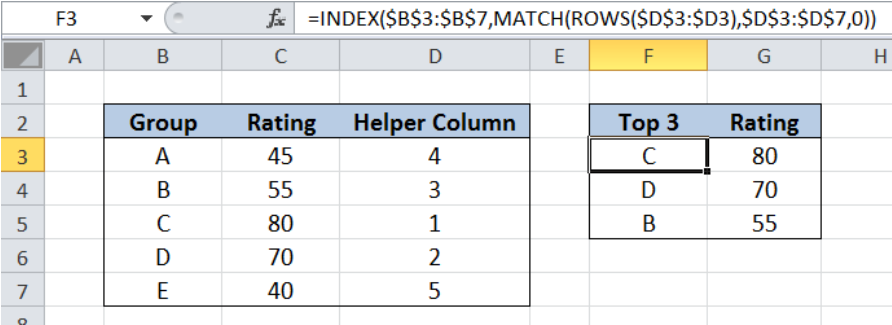Figure 1. Final result: Display sorted values with helper column

Formula for Top Group :  `=INDEX(\$B\$3:\$B\$7,MATCH(ROWS(\$D\$3:\$D3),\$D\$3:\$D\$7,0))`

Formula for Top Rating :  `=INDEX(\$C\$3:\$C\$7,MATCH(ROWS(\$D\$3:\$D3),\$D\$3:\$D\$7,0))`

## Syntax of the INDEX function

INDEX function returns a value from within a range, as specified by the row and column number

`=INDEX(array, row_num, column_num)`

The parameters are:

• array – a range of cells where we want to retrieve some data
• row_num – the row in the array from which we want to retrieve data
• column_num – the column in the array from which we want to retrieve data; if the array has only one column, column_num can be omitted

## Syntax of the MATCH function

MATCH function returns the position of a value in a range

`=MATCH(lookup_value, lookup_array, [match_type])`

The parameters are:

• lookup_value – a value which we want to find in the lookup_array
• lookup_array – the range of cells containing the value we want to match
• [match_type] optional; the type of match; if omitted, the default value is 1; We use 0 to find an exact match

## Syntax of the ROWS function

ROWS function returns the number of rows in a data set

`=ROW(array)`

• array– an array, range or reference for which we want to determine the number of rows

## Setting up Our Data

Our table contains three columns: Group (column B), Rating (column C) and a Helper Column (column D).  A helper column is a column we set-up to help simplify a set of data. In column D, we determine the rank of the groups by rating from 1 to 5, with 1 being the highest.

In column F, we want to find the top 3 groups in the order of 1, 2 and 3.  In column G, we want to find the corresponding rating for each of the top 3 groups.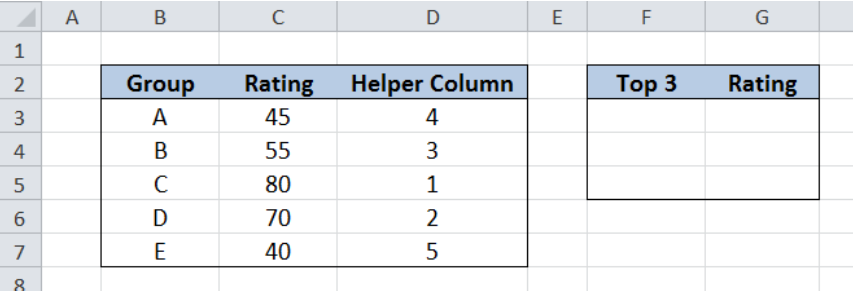Figure 2. Sample data to display sorted values with helper column

## Display top 3 groups

We want to identify the top 3 groups and display them in proper order in column F, with the help of our helper column (column D).  Let us follow these steps:

Step 1.  Select cell F3

Step 2.  Enter the formula: `=INDEX(\$B\$3:\$B\$7,MATCH(ROWS(\$D\$3:\$D3),\$D\$3:\$D\$7,0))`

Step 3:  Press ENTER

Step 4:  Copy the formula in cell F3 to cells F4:F5 by clicking the “+” icon at the bottom-right corner of cell F3 and dragging it down

The dollar signs “\$” in the formula fix the cells so that we can easily copy and paste the formula to other cells.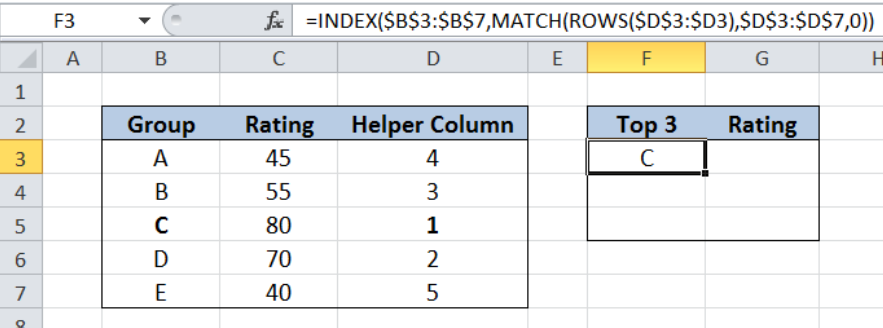Figure 3. Entering the formula using INDEX, MATCH and ROWS

Our array for the INDEX function is the range B3:B7.  Since there is only one column, we can omit the column_num in our formula.  The row_num is determined by the MATCH function : `MATCH(ROWS(\$D\$3:\$D3),\$D\$3:\$D\$7,0)`

Our lookup_value for the MATCH function is `ROWS(\$D\$3:\$D3)`, which returns the number of rows of D3:D3, whose value is 1. Hence, we want to match the value 1 in the lookup_array D3:D7 `{4;3;1;2;5}`. The value 1 is the third value in the array so the MATCH function returns 3.

Finally, the INDEX function returns the third row in the range B3:B7, which is B5, or “C”.  The result displayed in cell F3 is group C, which is also the top 1 group with the highest rating.

Copying the formula to cells F4 and F5 will return the top 2 and top 3 groups.  Below table displays the top 3 groups sorted according to rating.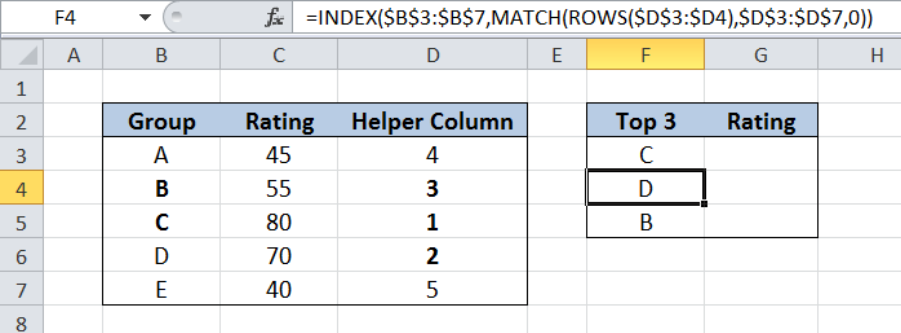Figure 4. Output:  Display top 3 groups sorted from highest rating

## Display top 3 ratings

Now that we’ve identified the top 3 groups, we want to find the corresponding ratings.  The formula is quite similar to our previous example. We only have to change the array for our INDEX function to the range C3:C7, instead of B3:B7.

Step 1.  Select cell G3

Step 2.  Enter the formula: `=INDEX(\$C\$3:\$C\$7,MATCH(ROWS(\$D\$3:\$D3),\$D\$3:\$D\$7,0))`

Step 3:  Press ENTER

Step 4:  Copy the formula in cell G3 to cells G4:G5 by clicking the “+” icon at the bottom-right corner of cell G3 and dragging it down

Our formula returns the value in column C based on the row number given by the MATCH and ROWS functions.

Below table displays the ratings of the top 3 groups.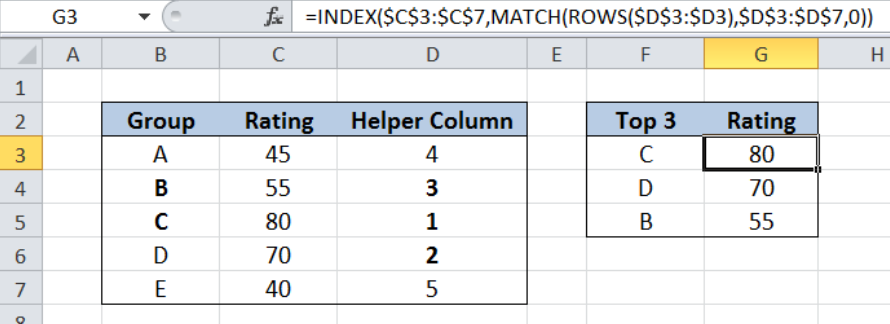Figure 5. Output:  Display top 3 ratings sorted from highest value

Most of the time, the problem you will need to solve will be more complex than a simple application of a formula or function. If you want to save hours of research and frustration, try our live Excelchat service! Our Excel Experts are available 24/7 to answer any Excel question you may have. We guarantee a connection within 30 seconds and a customized solution within 20 minutes.

### Did this post not answer your question? Get a solution from connecting with the expert.Another blog reader asked this question today on Excelchat:
Solution examplesindex and match with duplicates. I need to use another column as a reference, so my return value has two match the value of two things for it to return
Solved by E. H. in 60 minsHello, I have a big spreadsheet in which I need to know how many patients came in each month based on provider. I am using the following formula but I still get the N/A error. =INDEX('No Show Appts Data'!D:E, MATCH(1, 'No Show Appts Data'!D:D='No Shows Data'!M2)*('No Shows Data'!E:E='No Shows Data'!N1),0)) No Show Appts Data is the name of the sheet where Column D is Month Year of date in question and Column E is the provider. No Shows Data is the sheet where I am making all the formula calculation where Column M is Month and Year and Column N is the provider in question and therefore M2 is the month in question and N1 the provider in question. How do I fix this error? Thanks
Solved by F. H. in 40 minsI need to find an INDEX function that will convert the Call Day (a number 1-7) to the actual weekday found in row 1 of the DayofWeek named range. I don't know if I'm supposed to use the MATCH function as well or not.
Solved by D. D. in 11 minsI have two columns with names and a third one with email addresses. For every name that matches, I need to copy the email address to an empty column next to the matching name. .
Solved by E. U. in 15 minshelp with INDEX(IndexArea,MATCH(\$M\$5,MatchRows,0),MATCH(\$M\$6,MatchColumns,0))
Solved by Z. Y. in 12 mins## Subscribe to Excelchat.coAnother blog reader asked this question today on Excelchat: